# Adding & Subtracting 2-Digit NumbersTimes up!
Times up! You may finish answering the current question and then proceed to your score!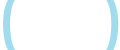Sorry, you have used all of your available hints for this lesson.
Practice Limit Reached

You've reached your daily practice limit of 12 questions.

Award InformationAwarded toQuestion Hint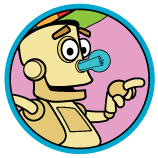Choose Family Member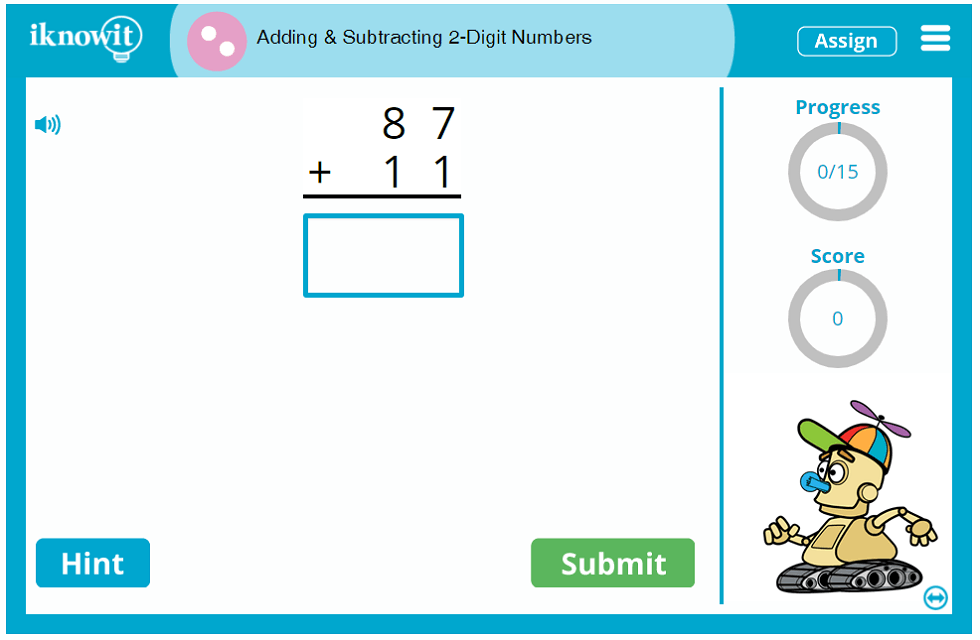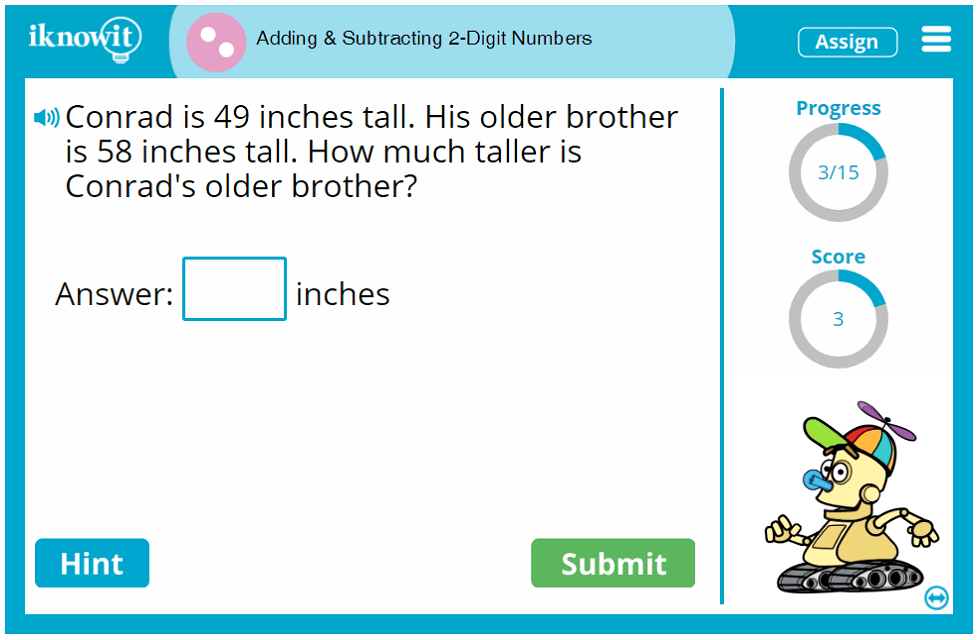Ready to give your students addition and subtraction practice in an engaging, digital format? Try out this adding and subtracting activity from iKnowIt.com! Here are the learning objectives for this interactive addition and subtraction activity geared toward second grade:

• Students will use their knowledge of place value and the properties of operations to answer a variety of addition and subtraction problems.
• Students will solve vertical addition problems, horizontal addition problems, addition word problems, vertical subtraction problems, horizontal subtraction problems, and subtraction word problems.
• Students will identify whether a word problem requires them to add or subtract.
• Students will practice addition and subtraction with two-digit numbers, including regrouping.

When your second grade students practice addition and subtraction skills in this online math game, they will have access to some awesome built-in math lesson features designed to help them make the most of their math practice session. For example, children can click on the "Hint" button to view a custom clue for the math problem. The hint consists of a written or pictorial clue that points students in the right direction without giving away the answer. When students answer a question incorrectly, a detailed explanation page will show them the correct answer, accompanied by an easy-to-understand explanation. This way, students can learn from prior mistakes as they progress through the interactive math activity.

In addition, this second grade mixed addition and subtraction activity comes with other handy features that enhance the math practice experience for kids. A progress-tracker in the upper-right corner of the practice screen shows students how many questions they have answered out of the total number of questions in the math lesson. A score-tracker beneath that lets students know how many points they have earned for correct answers so far. Opposite, a speaker icon indicates the read-aloud feature; students can click the button to have the question read out loud to them in a clear voice. This resource is ideal for ESL/ELL students and children who excel as auditory learners. Each of the I Know It lesson features is designed with your students' math achievement in mind.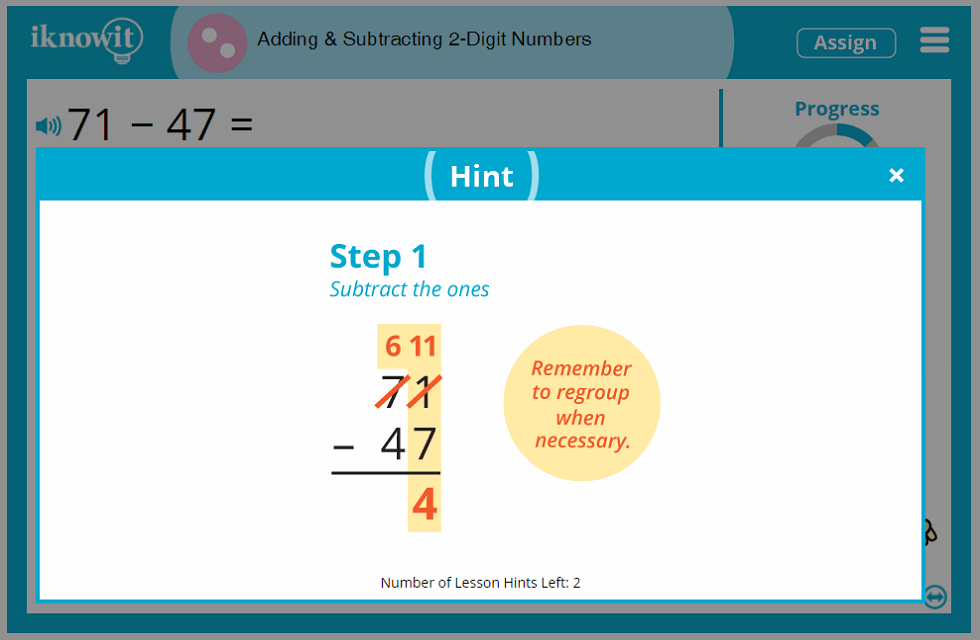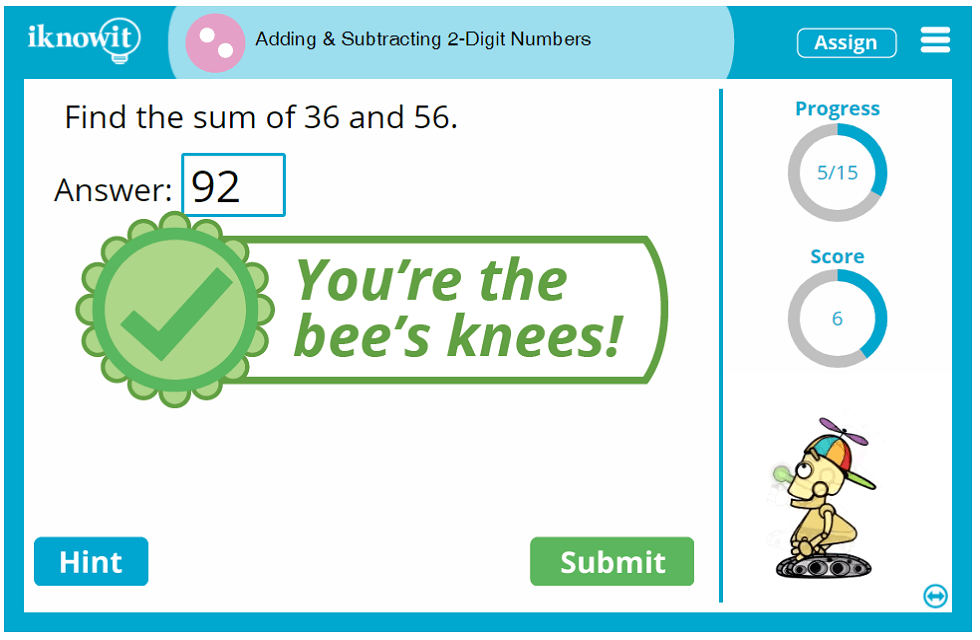## Teachers and Students Love Digital Math Games

Elementary educators enjoy using iKnowIt.com to help their students practice the math skills they are learning in class. When used alongside a comprehensive math curriculum, I Know It is a fantastic math practice resource that encourages little ones to sharpen their math skills in an interactive, digital format. Teachers appreciate the many math topics covered by the I Know It math lesson collection. Find dozens of math lessons from kindergarten through fifth grade, all written by accredited elementary math teachers and aligned to the Common Core Standard. Math activities are organized on the website by grade level and topic, making it easy for you to find and assign math lessons in a few clicks.

We hope you and your class will have a lot of fun trying out this second grade mixed addition and subtraction activity. Be sure to explore the hundreds of second grade math lessons in our online collection too.

## Discover the Benefits of an I Know It Membership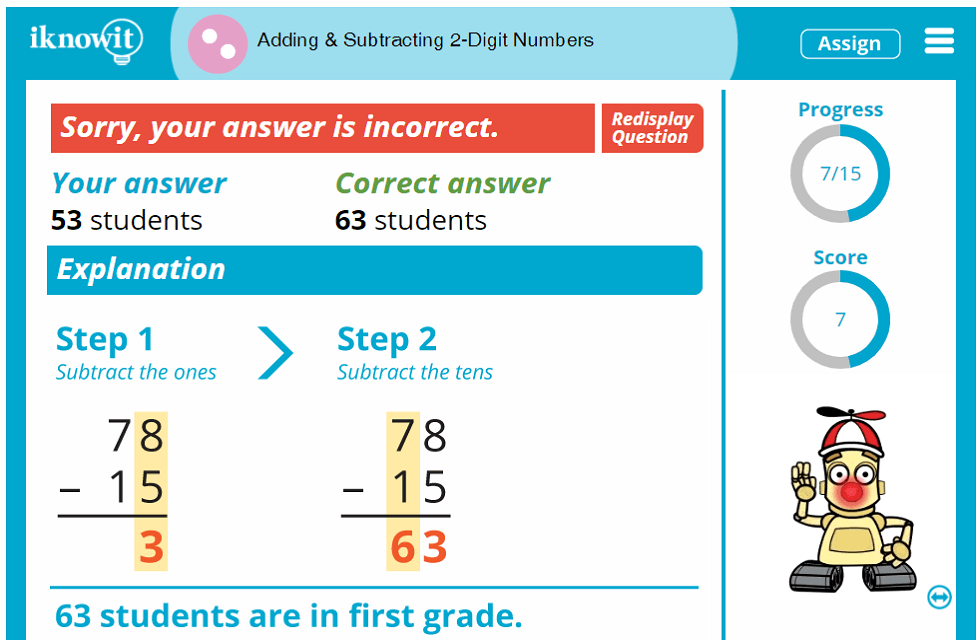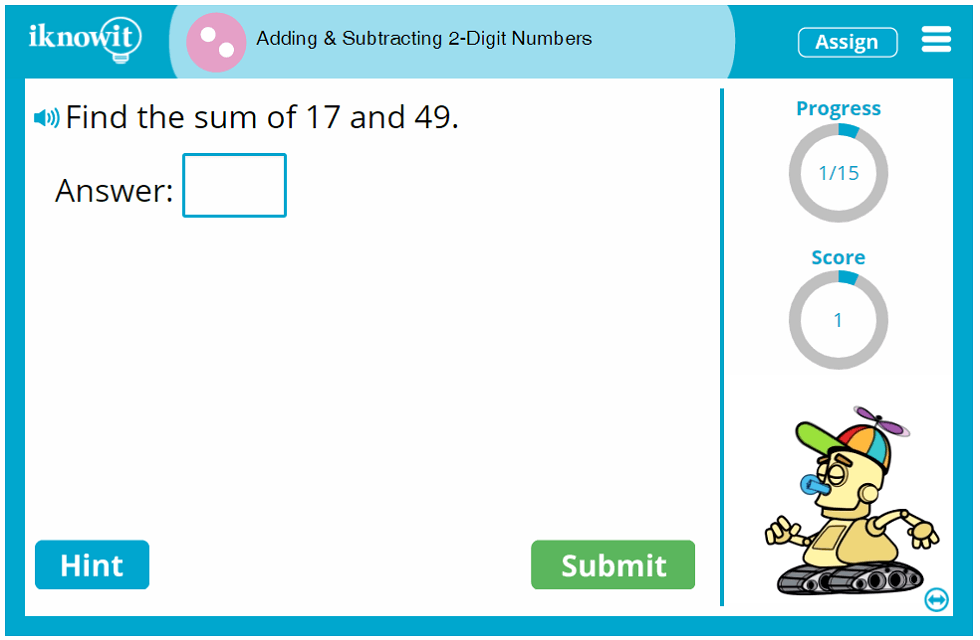## Level

This interactive math lesson is classified as Level B. It may be ideal for second grade students.

## Common Core Standard

2.NBT.5
Numbers and Operations in Base Ten

Students will use their understanding of place value and the properties of operations to add and subtract. Students will fluently add and subtract within one hundred using strategies based on place value, properties of operations, and/or the relationship between addition and subtraction.

## You might also be interested in...

Add or Subtract 10 or 100 (Level B)
In this second grade-level math lesson, students will practice adding or subtracting ten or one hundred. Questions are presented as vertical addition problems, horizontal addition problems, addition word problems, vertical subtraction problems, horizontal subtraction problems, and subtraction word problems.

Tables: Addition and Subtraction within 100 (Level B)
Students will practice adding and subtracting within one hundred in this online math activity geared toward second grade. Questions are presented as In/Out tables for which students must fill in the blank.